# An introduction to Generative Adversarial Networks (GANs), a semi-supervised learning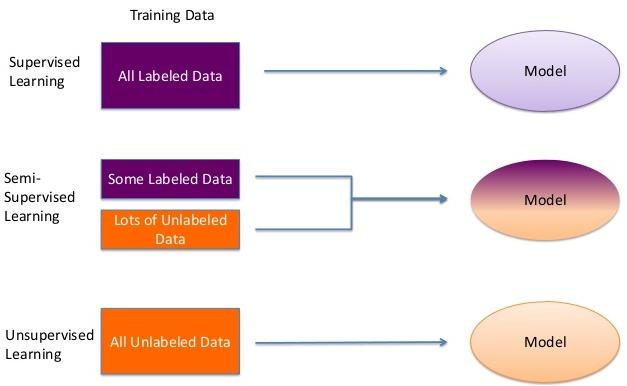# What is semi-supervised learning?

As you know, Data scientists use many different kinds of machine learning algorithms to discover patterns in big data that lead to actionable insights. At a high level, these different algorithms can be classified into two groups based on the way they “learn” about data to make predictions: supervised and unsupervised learning.

As you may have guessed, semi-supervised learning algorithms are trained on a combination of labeled and unlabeled data. This is useful for a few reasons. First, the process of labeling massive amounts of data for supervised learning is often prohibitively time-consuming and expensive. What’s more, too much labeling can impose human biases on the model. That means including lots of unlabeled data during the training process actually tends to improve the accuracy of the final model while reducing the time and cost spent building it.

As a result, semi-supervised learning is a win-win for use cases like webpage classification, speech recognition, etc. The goal is to combine these sources of data to train a Deep Convolution Neural Networks (DCNN) to learn an inferred function capable of mapping a new datapoint to its desirable outcome.

# Introduction to GAN

GANs are a kind generative models designed by Goodfellow et all in 2014. In a GAN setup, two differentiable functions, represented by neural networks, are locked in a game. The two players, the generator and the discriminator, have different roles in this framework.

The generator trying to maximize the probability of making the discriminator mistakes its inputs as real.

The discriminator guiding the generator to produce more realistic images.

In the perfect equilibrium, the generator would capture the general training data distribution. As a result, the discriminator is always unsure of whether its inputs are real or not.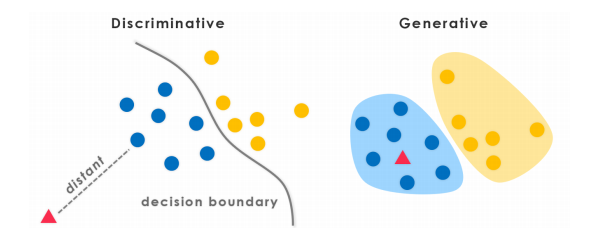### GAN structure

Assuming that we are working with an image dataset and as mentioned above, Generative adversarial networks are composed of 2 deep networks:

• Generator (G): A deep network generates realistic images.
• Discriminator (D): A deep network distinguishes real images from computer generated images.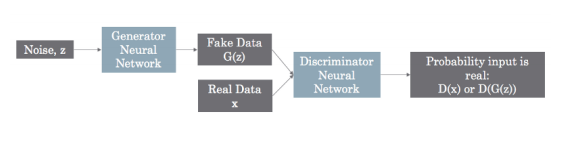The Generator is a neural network `G(z, θ1)`. It’s role is to map input noise variables z to the desired data space x (say images). The Discriminator is a second neural network `D(x, θ2)` that outputs the probability that the data came from the real dataset, in the range (0,1).

In both cases, `θi` represents the weights or parameters that define each neural network.

The Discriminator is trained to correctly classify the input data as either real or fake. It’s weights are updated as to maximize the probability that any real data input x is classified as the real dataset, while minimizing the probability that any fake image is classified as the real dataset. In more technical terms, the loss/error function uses maximizes the function `D(x)`, and it also minimizes `D(G(z))`.

The Generator is trained to fool the Discriminator by generating data as realistic as possible. The Generator’s weight’s are optimized to maximize the probability that any fake image is classified as the real datase. Formally this means that the loss/error function used for this network maximizes `D(G(z))`.

### GAN application

GANs are one of the hottest subjects in machine learning right now. These models have the potential of unlocking unsupervised learning methods that would expand ML to new horizons. It is being applied into those applications:

Generating data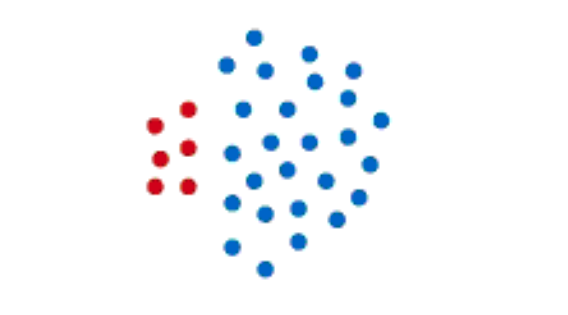Text translation into images.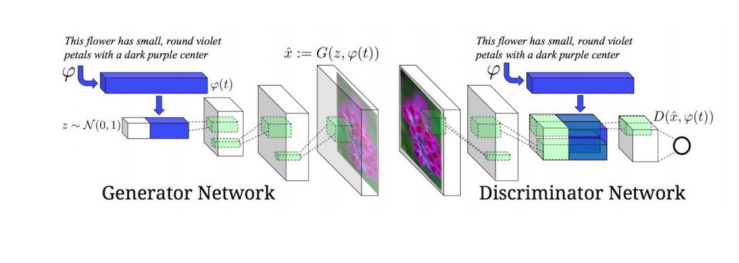Drug discovery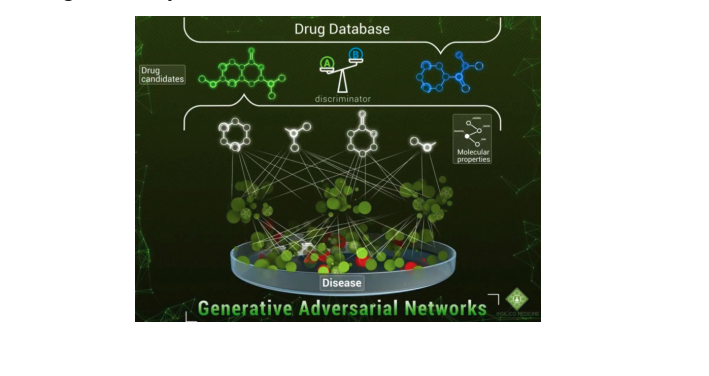# Conclusion

Generative Adversarial Networks are a recent development and have shown huge promises already. It is an active area of research and new variants of GANs are coming up frequently.

*References: *